Geometry

Length and Area: Level 1 Challenges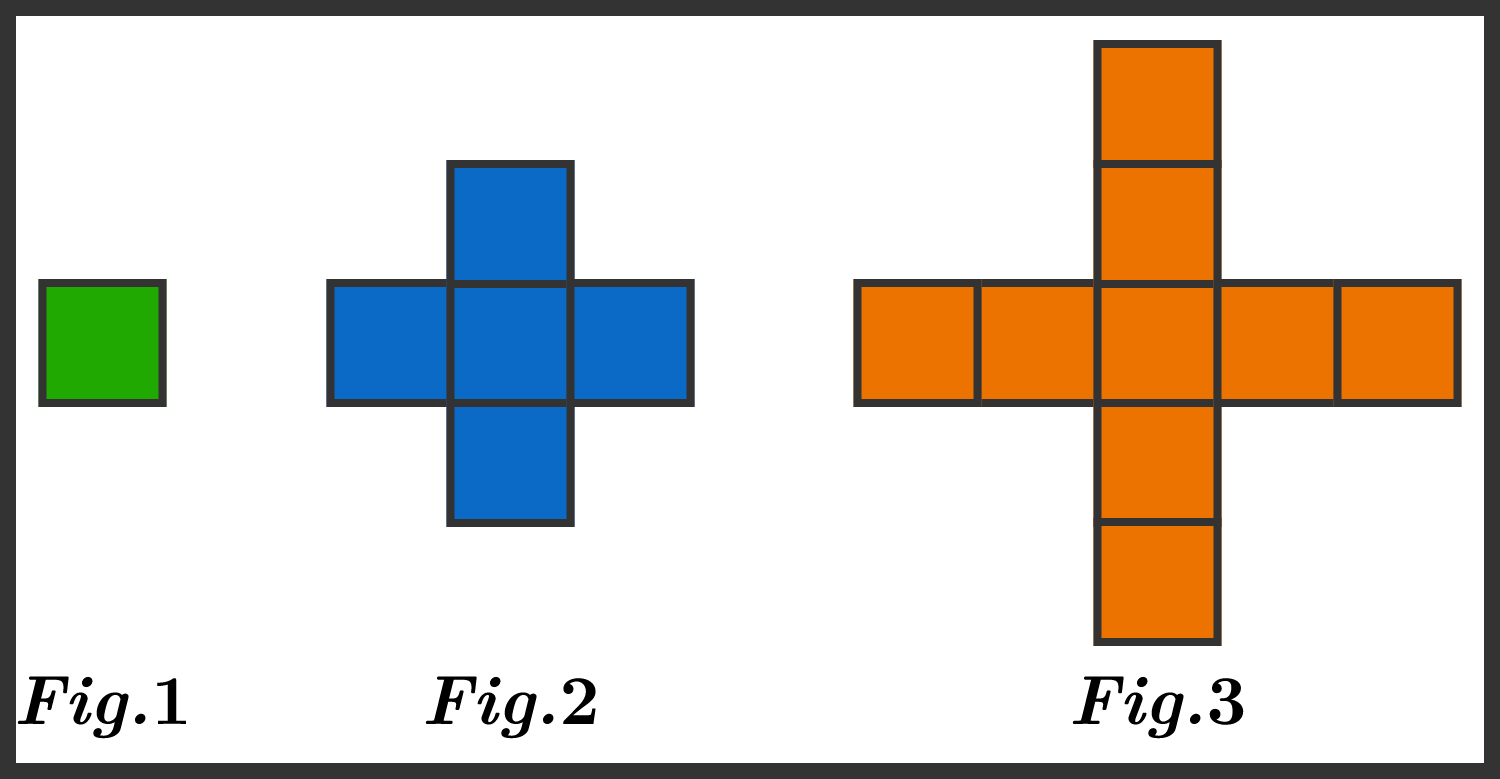By tracing the edges, we can calculate that the perimeter of figure 1 is 4 units, and the perimeter of figure 2 is 12 units. What is the perimeter of figure 3?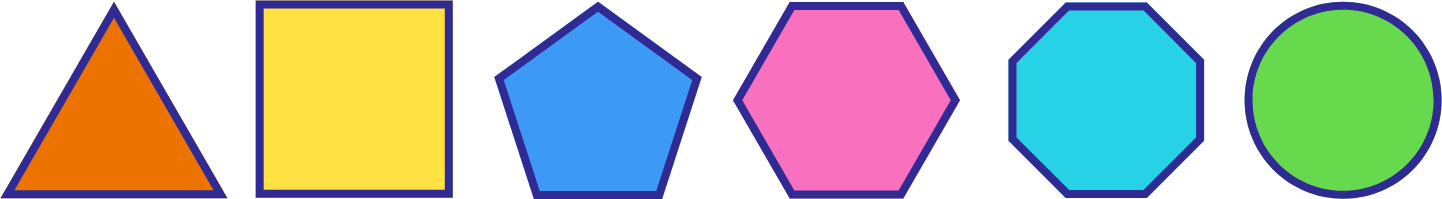A triangle, a square, a pentagon, a hexagon, an octagon, and a circle all have the same perimeter.

Which one has the smallest area?

Note: All of the polygons are regular.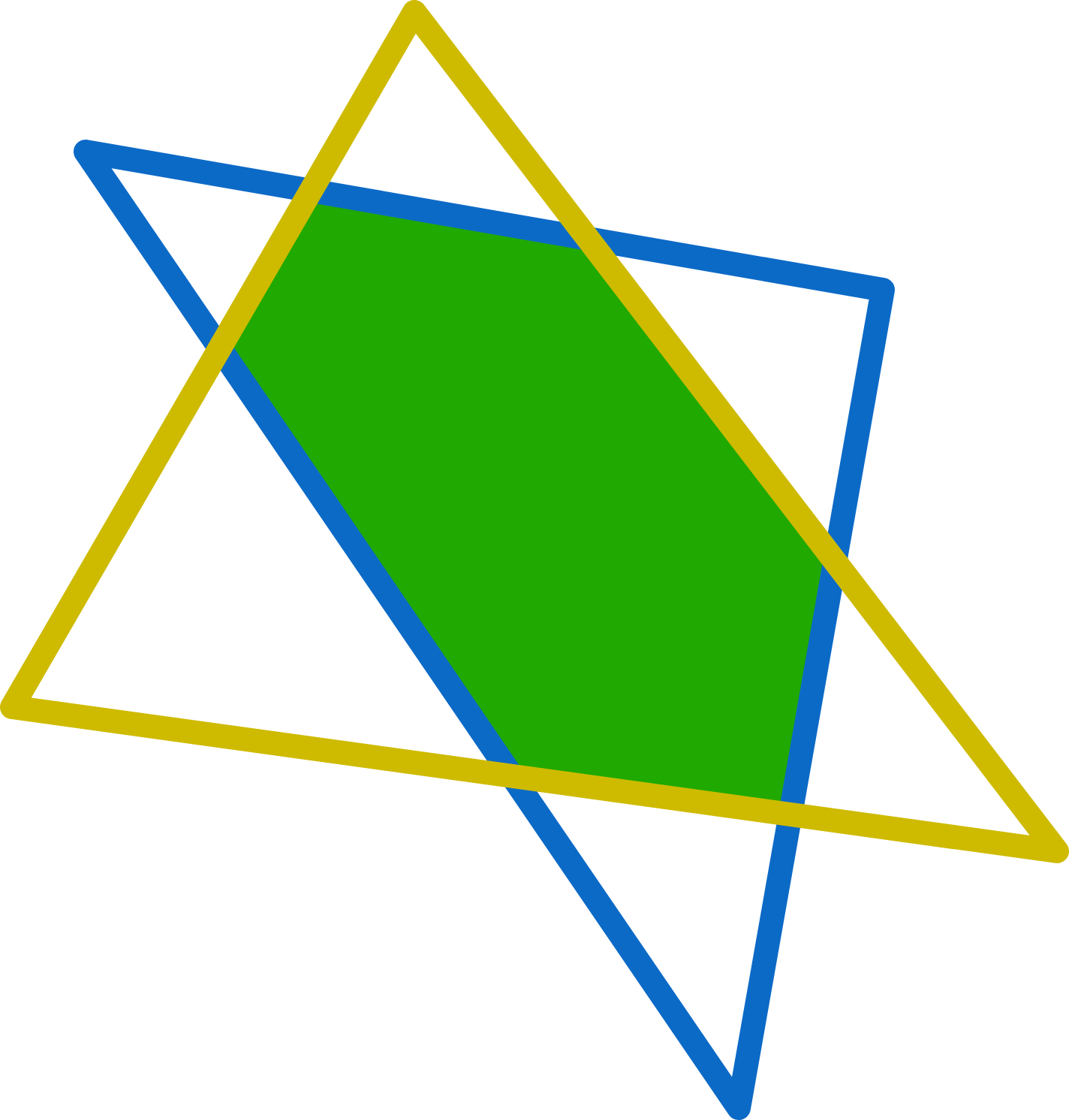If the perimeter of the yellow triangle is 20,
and the perimeter of the blue triangle is 30,
and the perimeter of the six-pointed star is 40,
what is the perimeter of the green hexagon?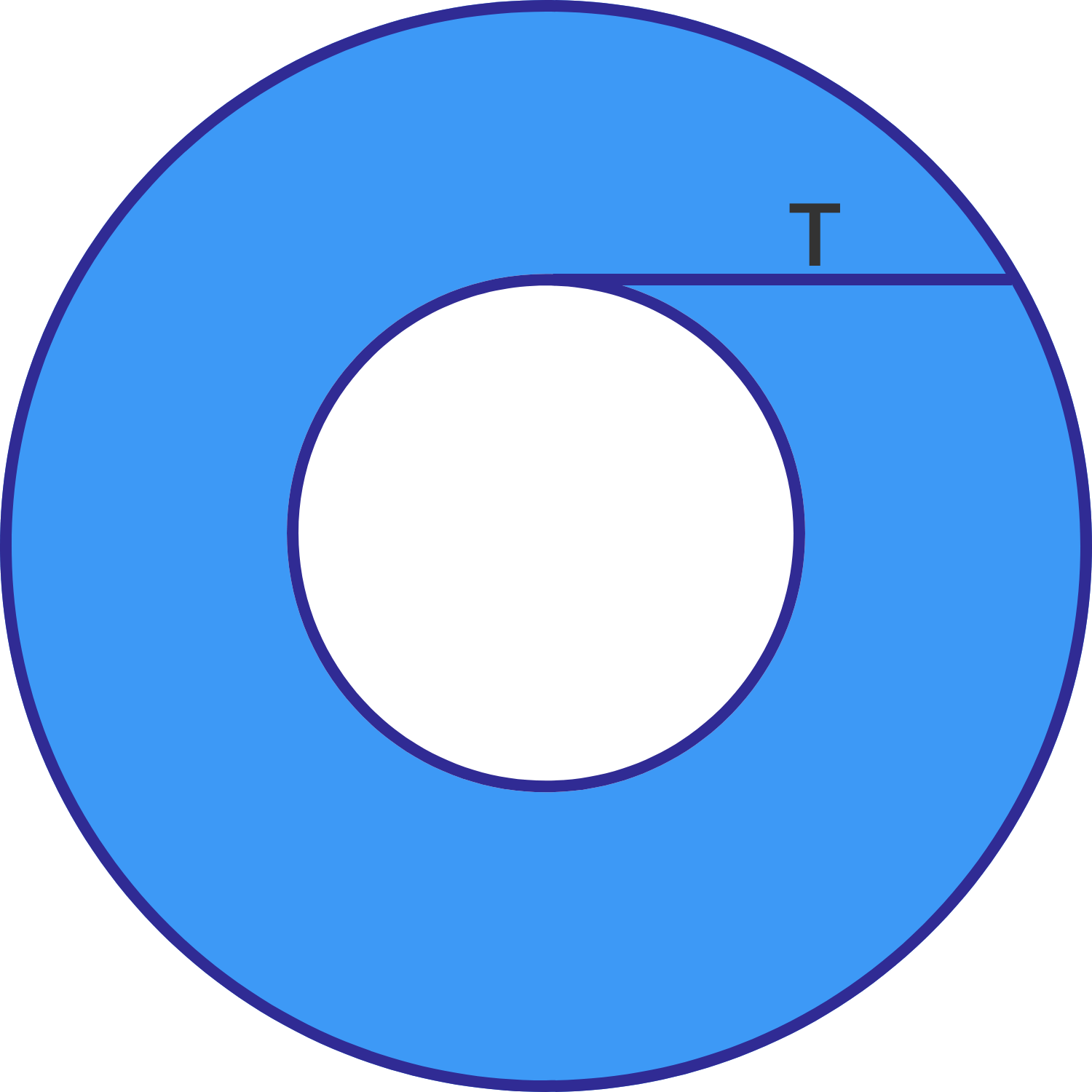Find the area of the shaded annulus.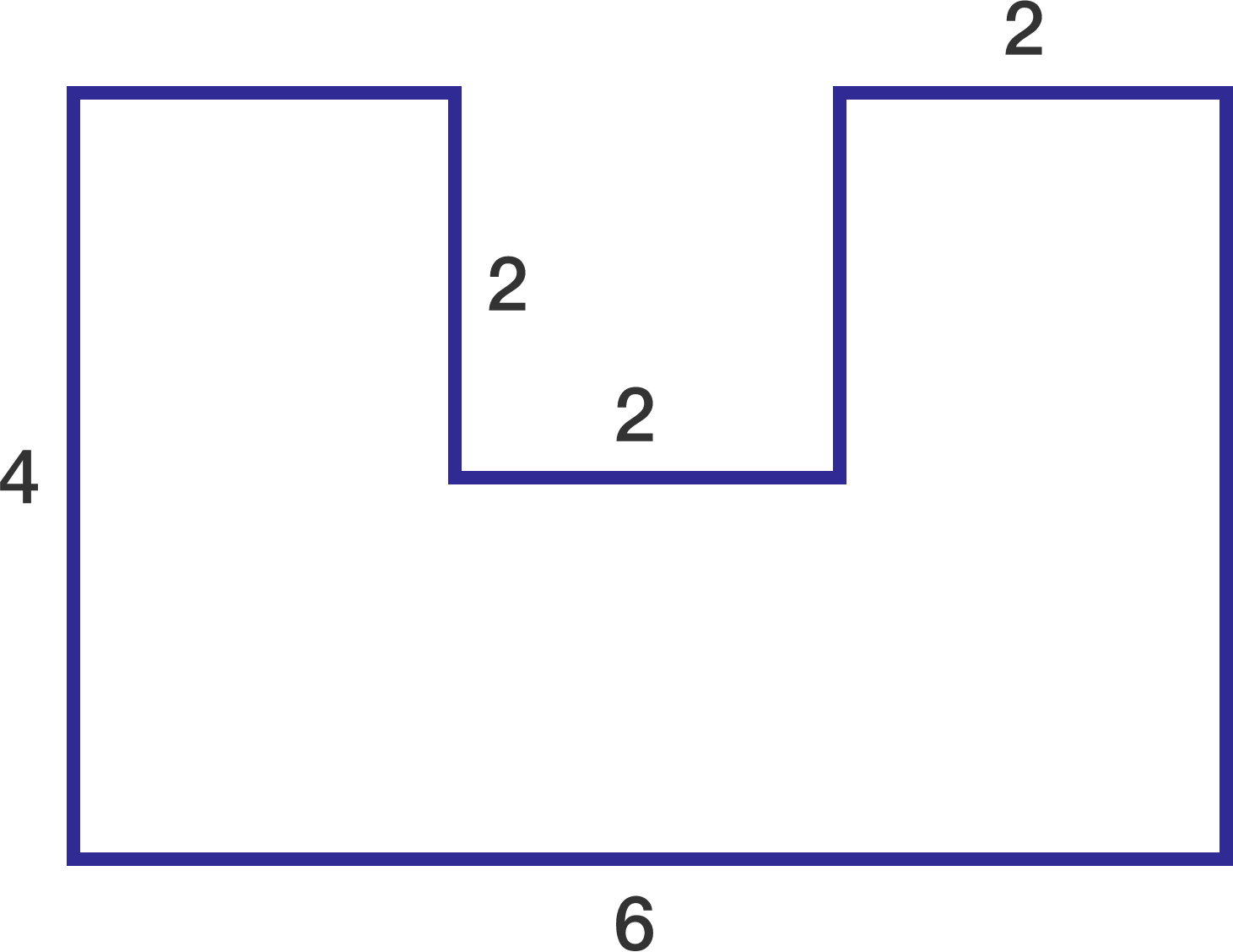What is the area of the given figure?

×